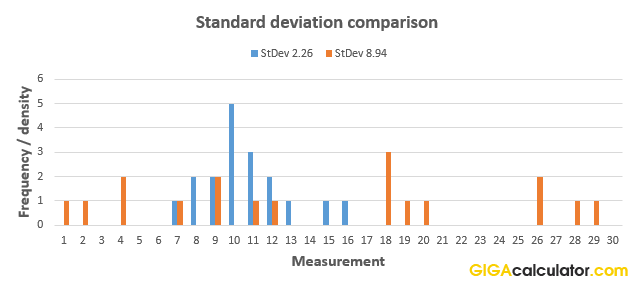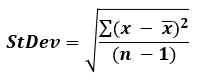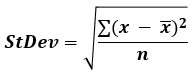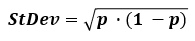# Standard Deviation Calculator

Use this calculator to easily calculate the standard deviation of a sample, or to estimate the population standard deviation based on a random sample from it. Standard deviation for binomial data. The calculator will also output the variance, arithmetic mean (average), range, count, and standard error of the mean (SEM).

### Calculation results

2.2608 Standard deviation Variance Mean SEM Range Count of numbers
Share calculator:

Embed this tool:
get code

## What is standard deviation?

Standard deviation is a term in statistics and probability theory used to quantify the amount of dispersion in a numerical data set, that is - how far from the normal (average) are the data points of interest. "Standard deviation" is often concatenated to SD or StDev and is denoted by the Greek letter sigma σ when referencing a population estimate based on a sample and the small Latin letter s when referencing sample standard deviation which is directly calculated.

A low standard deviation σ means that the data points are clustered around the sample mean while a high SD indicates that the set of data is spread over a wide range of values. The graph below illustrates the point by comparing two distributions of 18 elements each, with different standard deviations (2.26 and 8.94):You can check the numbers in the data sets and the resulting calculations in our SD calculator here for set 1 and here for set 2.

## How to calculate standard deviation

Standard deviation is calculated as the square root of the variance, while the variance itself is the average of the squared differences from the arithmetic mean. We square the differences so that larger departures from the mean are punished more severely, and it also has the side effect of treating departures in both directions (positive errors and negative errors) equally. The standard deviation is preferred over the variance when describing statistical data since it is expressed in the same unit as the values in the data. Our stdev calculator also calculates the variance for you.

For continuous outcome variables you need the whole raw dataset, while for binomial data - proportions, conversion rates, recovery rates, survival rates, etc. you can calculate the variance and standard deviation using just two summary statistics: the number of observations (sample size) and the rate of events of interest (which is also a mean). Our standard deviation calculator supports both continuous and binomial data. Equations for calculating standard deviation are presented below.

## Standard deviation formula

There are two formulas you should use, depending on whether you are calculating the standard deviation based on a sample from a population or based on the whole population.

To find the standard deviation from a sample, the sample standard deviation formula applies, which is:If the set of data represents the whole population of interest, find the standard deviation using the formula:In the population standard deviation formula above, x is a data point, x (read "x bar") is the arithmetic mean, and n is the number of elements in the data set (count). The summation is for the standard i=1 to i=n sum. As noted, the standard deviation is in both cases equal to the square root of the variance. Our standard deviation calculator supports both formulas with the flip of a switch.

In most cases you will find yourself using the sample standard deviation formula, as most of the time you will be sampling from a population and won't have access to data about the whole population. The formula our calculator uses in this case is known as the "corrected sample standard deviation" and it is not unique as unlike the sample mean and variance, there is no single formula that is an optimal estimator across all distributions. This formula, for example, can be heavily biased for n < 10.

In certain cases, you will have information about the whole population, for example if the population of interest is students in a class or a school, at a given time, it is possible to have the grades for all of them. However, most often the population of interest will span across time and cover too many individuals to be practically measured.

If you need to calculate the standard deviation for proportional data, event rates, etc. the formula is simply:where p is the proportion of the population that experiences the event of interest, or has a characteristic of interest. Since a proportion is just a special type of mean, this standard deviation formula is derived through a simple transformation of the above ones. Our standard deviation calculator supports proportions for which only the sample size and the event rate need to be known to estimate the difference between the observed outcome and the expected one.

## How to interpret the standard deviation?

As already shown in the example above, a lower standard deviation means lower dispersion in a data set - the numbers are more clustered around the mean. This quality means that standard deviation measures and estimates can be used to denote the precision of measuring tools, instruments, or procedures in physics, medicine, biology, physiology, chemistry, and so on. It can be thought of as a measurement of uncertainty in the data - expected, known or accepted, depending on context.

In many situations you will be presented by a statistical cut-off point in standard deviations. These can be equated to percentiles - what percentage of cases lie x standards deviations from the expected value. Here are some key levels and percentile cut-offs:

Table of commonly used standard deviation cut-offs for normally distributed variables:

Common standard deviation cut-offs
Standard deviationPercentile (1-sided)Percentile (2-sided)
0.3186σ 50.00% 25.00%
0.5000σ 69.15% 38.29%
0.6745σ 75.00% 50.00%
0.8416σ 80.00% 60.00%
1.0000σ 84.13% 68.27%
1.2816σ 90.00% 80.00%
1.6448σ 95.00% 90.00%
1.9599σ 97.50% 95.00%
2.3263σ 99.00% 98.00%
2.5758σ 99.50% 99.00%
3.7190σ 99.99% 99.98%

So, if an observation is 1.645 standard deviations from the expected value, it is in the top 10-th percentile of the population of interest. 2-sided refers to the direction of the effect you are interested in. In most practical scenarios the 1-sided number is the relevant one. In population studies, the 2-sided percentile is equivalent to the proportion within the bound specified by the standard deviation.

A geometrical interpretation would be that the standard deviation represents the portion of the area of a distribution that is included or excluded.

## Practical applications and examples

Standard deviations have an array of practical uses, most notably linked to statistics and measurements, which is why this online tool is in our "Statistics" category.

### Statistical inference

In statistical inference through null-hypothesis statistical tests the procedure is to establish what the expected distribution of outcomes from a test is, assuming a set of conditions are true, and then compare the actually observed data (converted to standard deviation measures) to that expected outcome. If the observed experimental data departs significantly from the expectation, there may be ground for inferring a breakthrough. Such results are often called "statistically significant". In statistical inference one deals with samples from a population, hence the sample standard deviation formula needs to be applied in order to estimate the population standard deviation.

Different practical situations require different thresholds (levels of statistical significance), which can be expressed in terms of standard deviations, say 2 standard deviations from the expected, or in terms of percentage probability of the observation under the null: 5%, 1%, etc. A value which is calculated as 1.96 standard deviations from the null cutoff will only be seen 5% of the time if the null hypothesis is in fact true. The number of standard deviations of an observation is often referred to as the Z-score. The experiments in CERN through which gravitational waves were discovered, for example, had a threshold of 6-sigma, so the observations from the experiment had to be extremely unlikely before a discovery was to be announced.

One reason the standard deviation of the mean (standard error of the mean, SEM) is the statistic of choice is that it is usually normally distributed, even if the underlying data is not. Thus, very often it is the mean of the experimental data which is compared to the expected mean and standard deviation of the mean, not individual data points.

### Finance

Standard deviation of the price fluctuations of a financial asset (stock, bond, property, etc.) is widely used to estimate the amount of risk of single assets or asset portfolios by financial managers and academic papers. This is, however, a hotly debated issue with many prominent financial practitioners denouncing the equation of risk and standard deviation. A popular technical analysis tool - the Bollinger Bands, is effectively plotting lines calculated so that they are two standard deviations in either direction from the mean price of a given rolling period.

Since standard deviation and other statistical tools only apply to stationary series, and some financial data is non-stationary, it needs to be transformed by removing trend, seasonality, and auto-correlation from the dataset, usually by way of differencing using complex regressions like ARIMA (AutoRegressive Integrated Moving Average) and exponential smoothing models.

### Weather

Standard deviation calculations often accompany climate data like mean daily maximum and minimum temperatures, as they help us understand how often and by how much they fluctuate. For example, coastal locations often have smaller temperature deviations when compared to inland locations, making the typical weather quite different, even if they have the same average temperature.

#### Cite this calculator & page

If you'd like to cite this online calculator resource and information as provided on the page, you can use the following citation:
Georgiev G.Z., "Standard Deviation Calculator", [online] Available at: https://www.gigacalculator.com/calculators/standard-deviation-calculator.php URL [Accessed Date: 29 May, 2023].